Download Presentation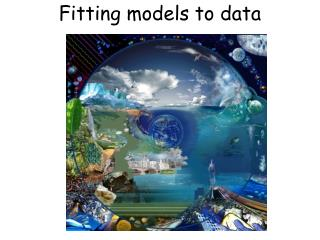Fitting models to data

# Fitting models to data - PowerPoint PPT Presentation

Fitting models to data. Step 5) Express the relationships mathematically in equations Step 6) Get values of parameters. Determine what type of model you will make - functional or mechanistic Use ”standard” equations if possible Analyse relationships with a statistical software.I am the owner, or an agent authorized to act on behalf of the owner, of the copyrighted work described.
Download Presentation## Fitting models to data

An Image/Link below is provided (as is) to download presentation

Download Policy: Content on the Website is provided to you AS IS for your information and personal use and may not be sold / licensed / shared on other websites without getting consent from its author.While downloading, if for some reason you are not able to download a presentation, the publisher may have deleted the file from their server.

- - - - - - - - - - - - - - - - - - - - - - - - - - E N D - - - - - - - - - - - - - - - - - - - - - - - - - -
Presentation Transcript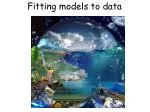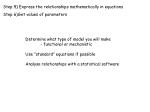Step 6) Get values of parameters

Determine what type of model you will make

- functional or mechanistic

Use ”standard” equations if possible

Analyse relationships with a statistical software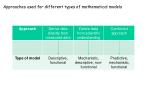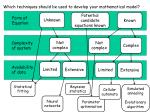Potential candidate equations known

Known

Unknown

Form of

Equation

Not complex

Complex

Not complex

Complexity of system

Extensive

Limited

Extensive

Limited

Availability of data

Statistical fitting

Bayesian statistics

Cellular automata

Simulated annealing

Evolutionary algorithm

Neural networks

Parameter optimisation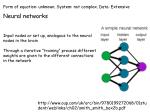Neural networks

Input nodes ar set up, analogous to the neural nodes in the brain

Through a iterative ”training” process different weights are given to the different connections in the network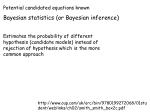Potential candidated equations known

Bayesian statistics (or Bayesian inference)

Estimates the probability of different hypothesis (candidate models) instead of rejection of hypothesis which is the more common approach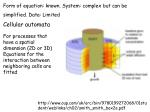Form of equation: known. System: complex but can be simplified. Data: Limited

Cellular automata

For processes that have a spatial dimension (2D or 3D)

Equations for the interaction between neighboring cells are fitted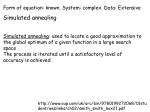Form of equation: known. System: complex. Data: Extensive

Simulated annealing

Simulated annealing: used to locate a good approximation to the global optimum of a given function in a large search space

The process is iterated until a satisfactory level of accuracy is achieved.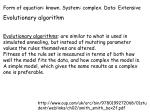Form of equation: known. System: complex. Data: Extensive

Evolutionary algorithm

Evolutionary algorithms: are similar to what is used in simulated annealing, but instead of mutating parameter values the rules themselves are altered.

Fitness of the rule set is measured in terms of both how well the model fits the data, and how complex the model is.

A simple model, which gives the same results as a complex one is preferable.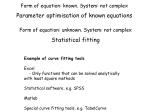Form of equation: known. System: not complex

Parameter optimisation of known equations

Form of equation: unknown. System: not complex

Statistical fitting

Example of curve fitting tools

Excel

- Only functions that can be solved analytically with least square methods

Statistical software, e.g. SPSS

Matlab

Special curve fitting tools, e.g. TabelCurveAdditive or multiplicative functions

Y = f(A) + f(B) Y 0-1

If equal weight: f(A) 0-0.5, f(B) 0-0.5

If f(A) = 0 and f(B) = 0 then y = 0

If f(A) = 0 and f(B) = 0.5 then y = 0.5

If f(A) = 0.5 and f(B) = 0 then y = 0.5

If f(A) = 0.5 and f(B) = 0.5 then y = 1

Multiplicative

Y = f(A) × f(B) Y 0-1

If equal weight: f(A) 0-1, f(B) 0-1

If f(A) = 0 and f(B) = 0 then y = 0

If f(A) = 0 and f(B) = 1then y = 0

If f(A) = 1 and f(B) = 0 then y = 0

If f(A) = 1 and f(B) = 1 then y = 1Example - stepwise fitting of a multiplicative function

Model of transpiration

Lagergren and Lindroth 2002Example - stepwise fitting of a multiplicative function

First try to find a theorethical base for the model

(r)

(DVPD)

(θ)

(T)

Lagergren and Lindroth 2002Example - stepwise fitting of a multiplicative function

Envelope fitting of the first dependency

(Alternately: Select a period when you expect no limitation from r, T or θ)

Gives: gmax and f(DVPD)Example - stepwise fitting of a multiplicative function

Select a period when you expect no limitation from T or θExample - stepwise fitting of a multiplicative function

Select a period when you expect no limitation from θExample - stepwise fitting of a multiplicative function

The remaining deviation should be explained by θExample - stepwise fitting of a multiplicative function

The modelled was controlled by applying it for the callibration year

And validated against a different year